# RD Sharma Solutions for Class 8 Maths Chapter 3 - Squares and Square Roots Exercise 3.9

Exercise 3.9 of RD Sharma Solutions for the Class 8 Maths Chapter 3, Squares and Square Roots. Students can refer and download from the links provided below. Our expert team at BYJU’S have solved the RD Sharma Solutions for Class 8 that help students practice the problems without any obstacles. Exercise 3.9 of Class 8 is about finding the approximate values of square roots by using square root tables. Practice RD Sharma Solutions for Class 8 for better understanding of the concepts, which help students obtain high marks in their examination.

## Download the pdf of RD Sharma Solutions For Class 8 Maths Chapter 3 Squares and Square Roots Exercise 3.9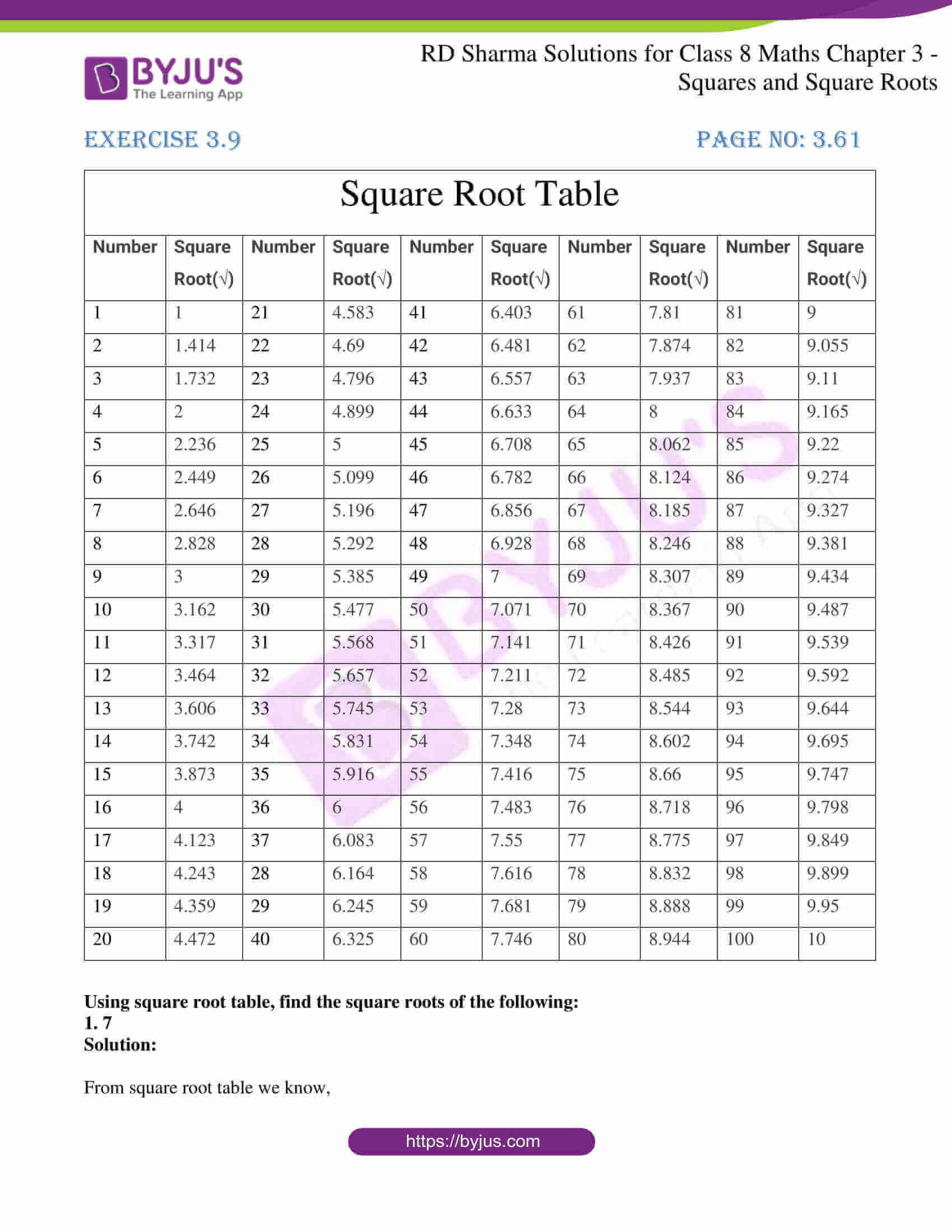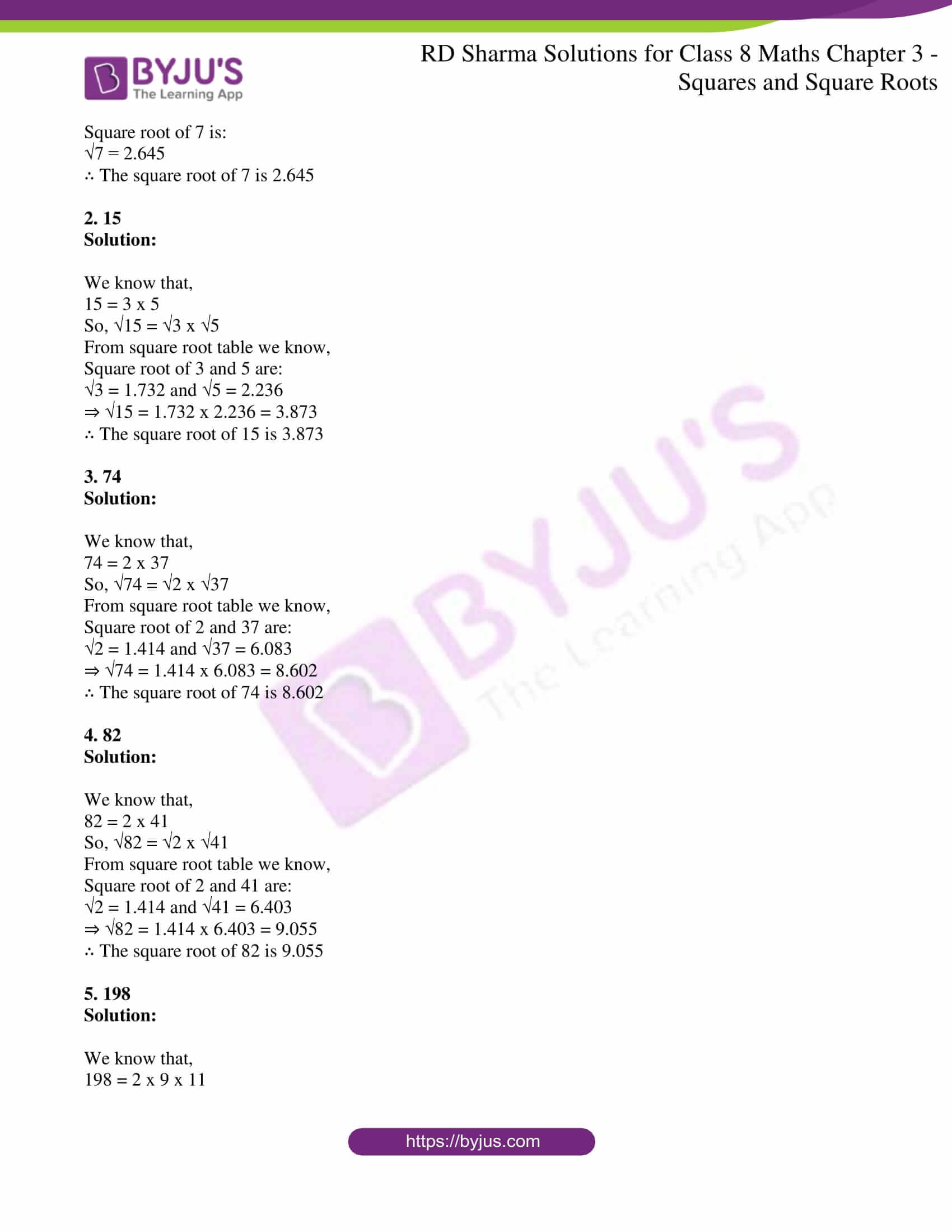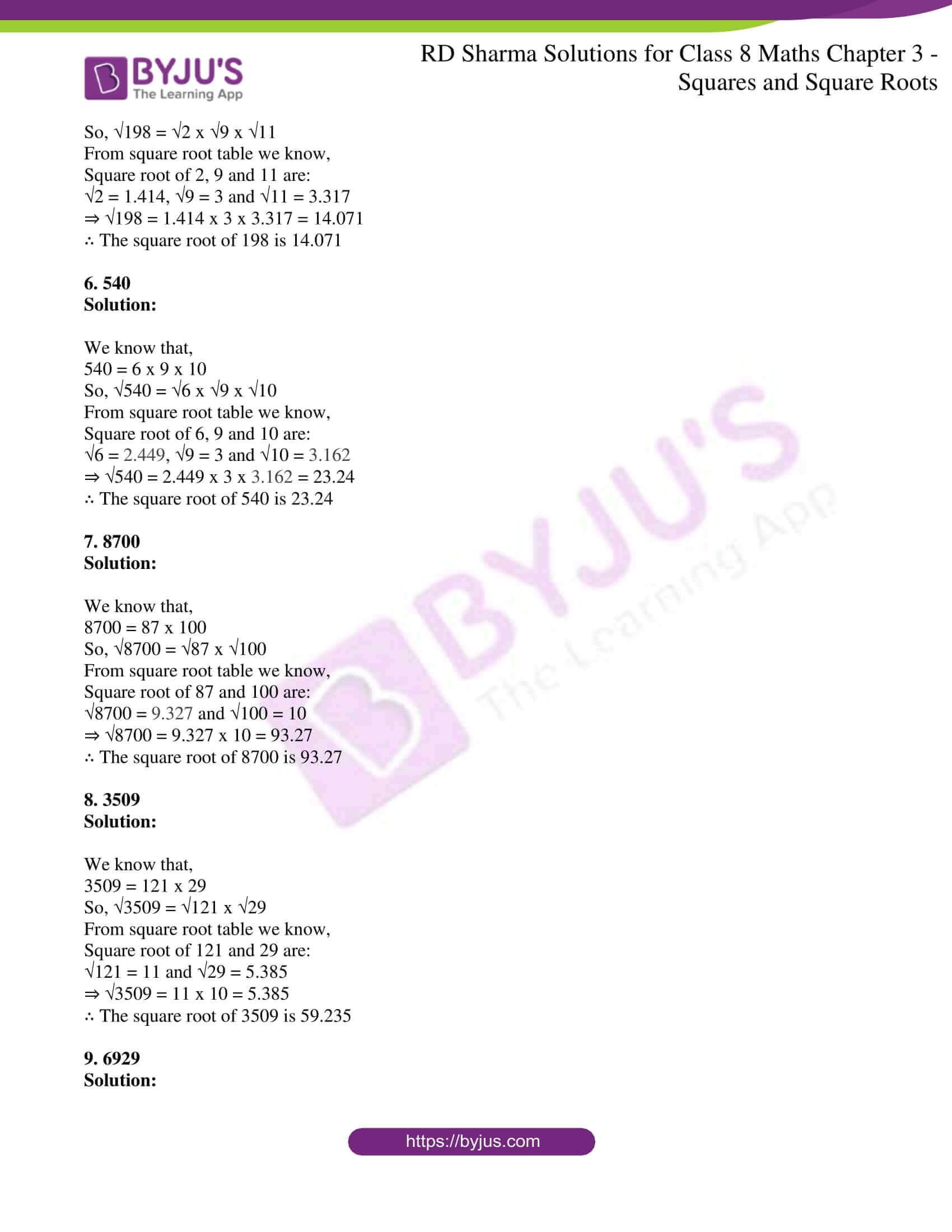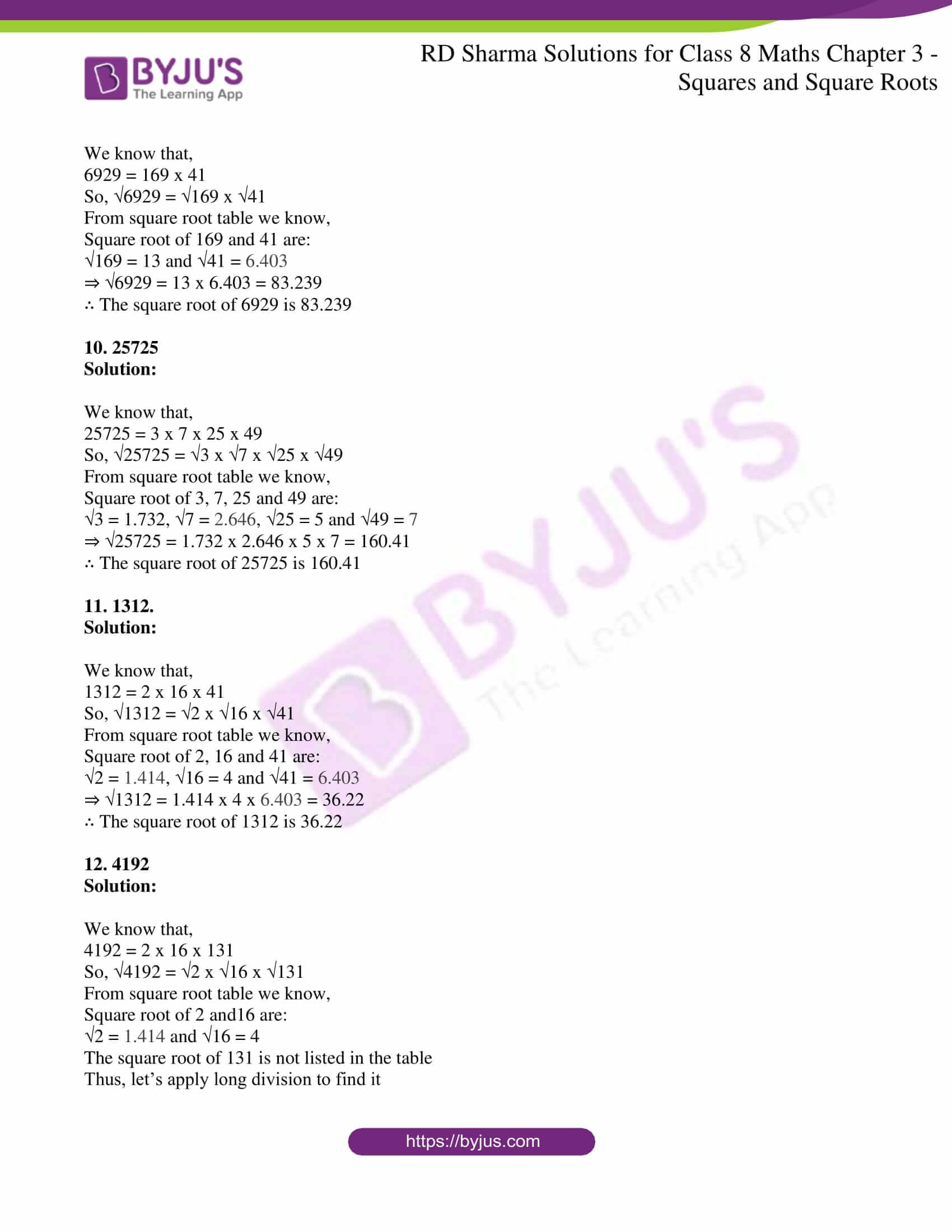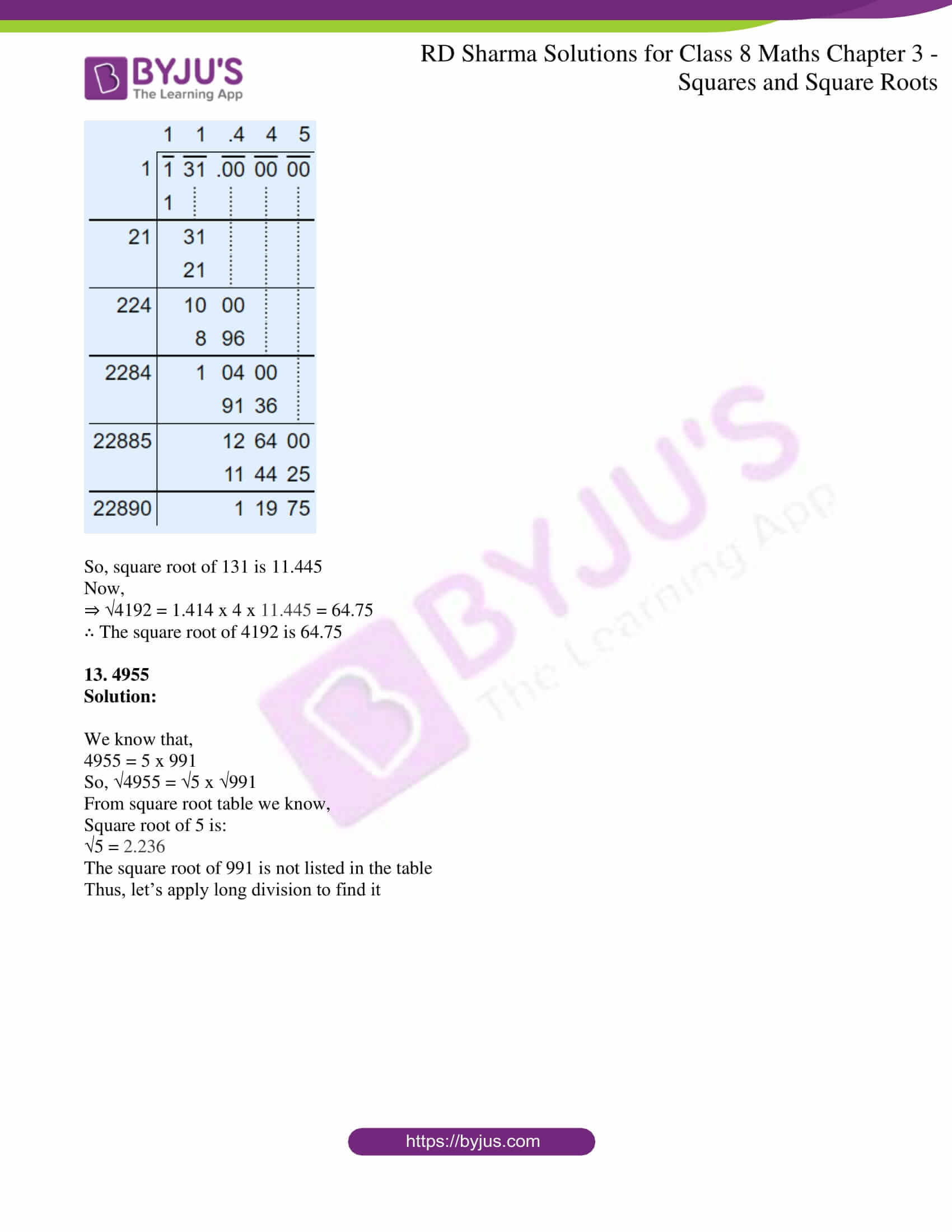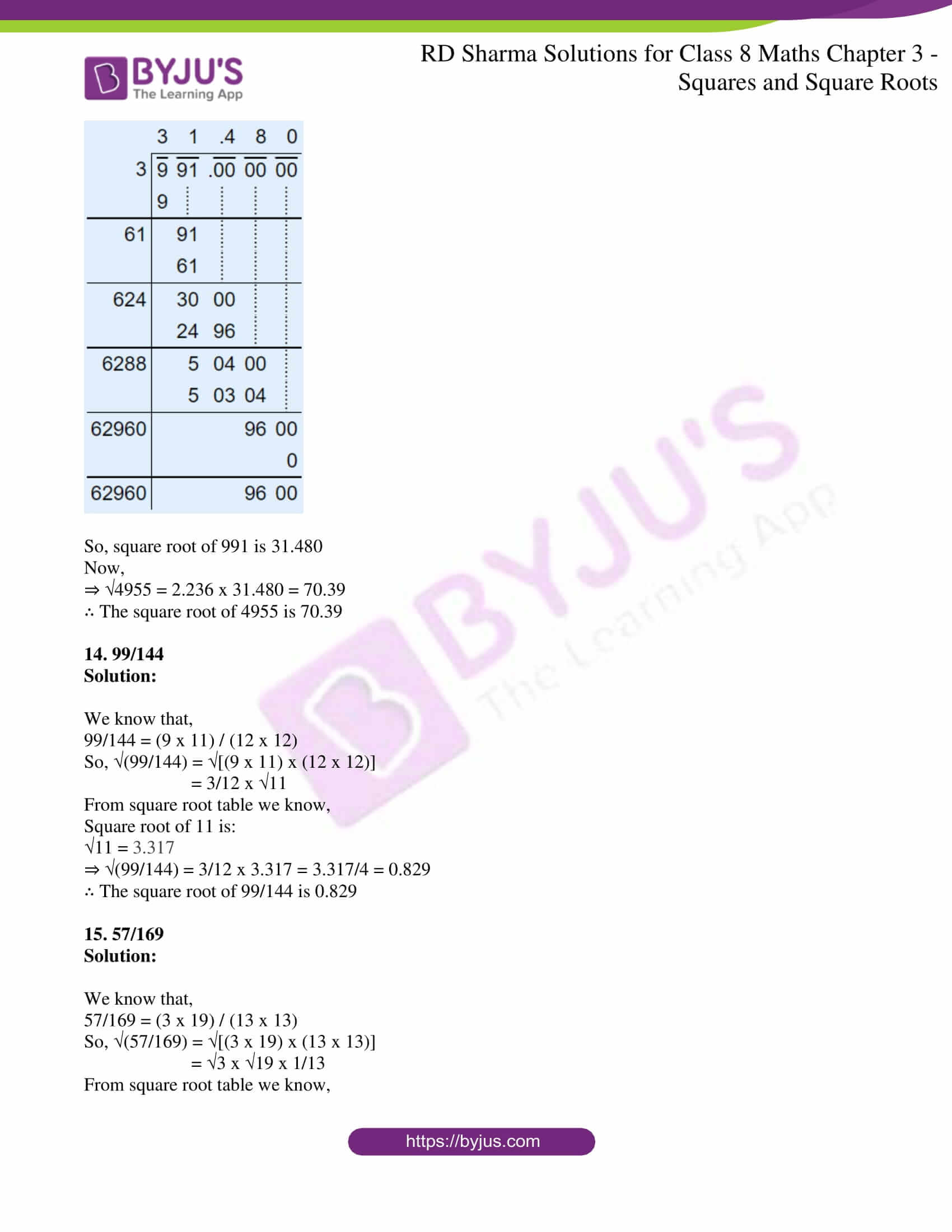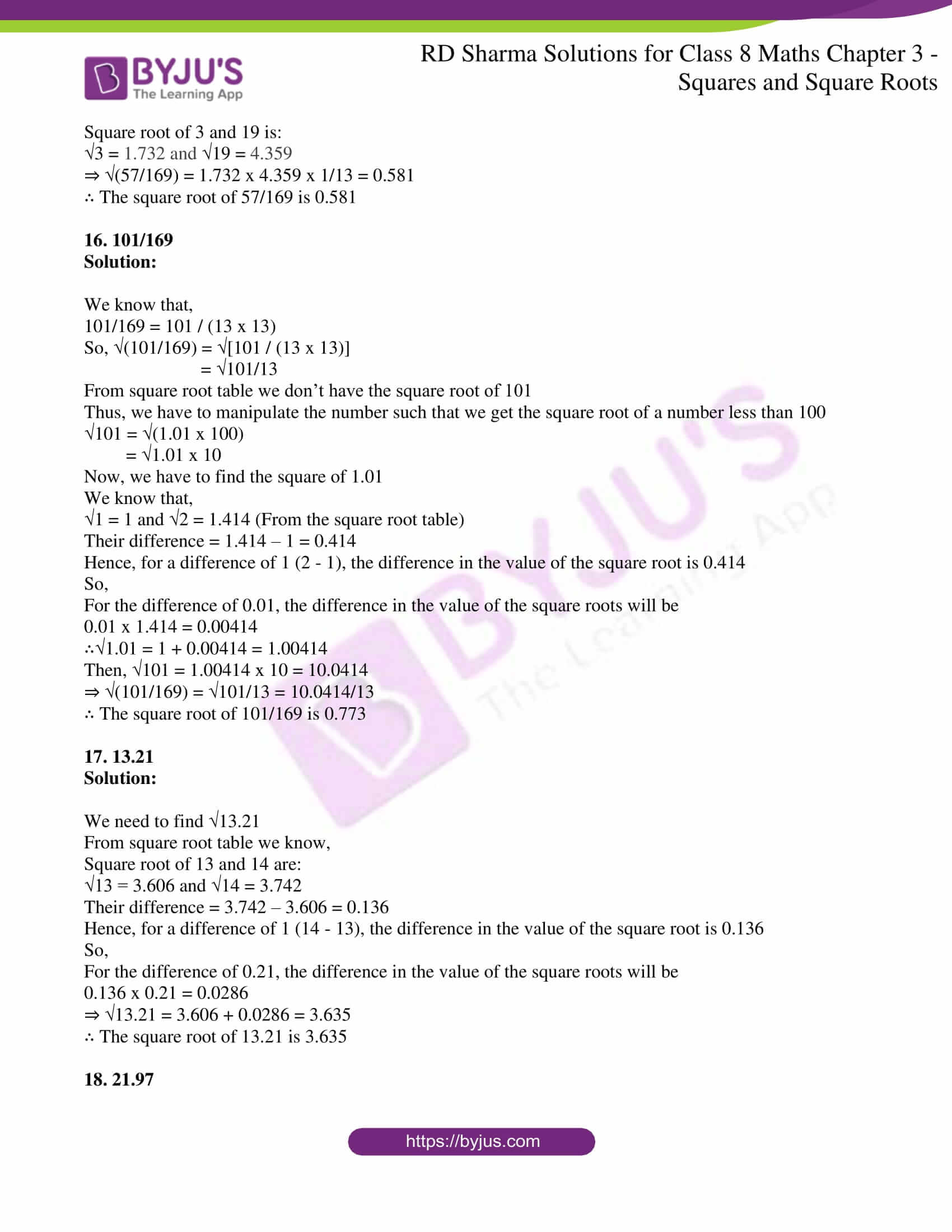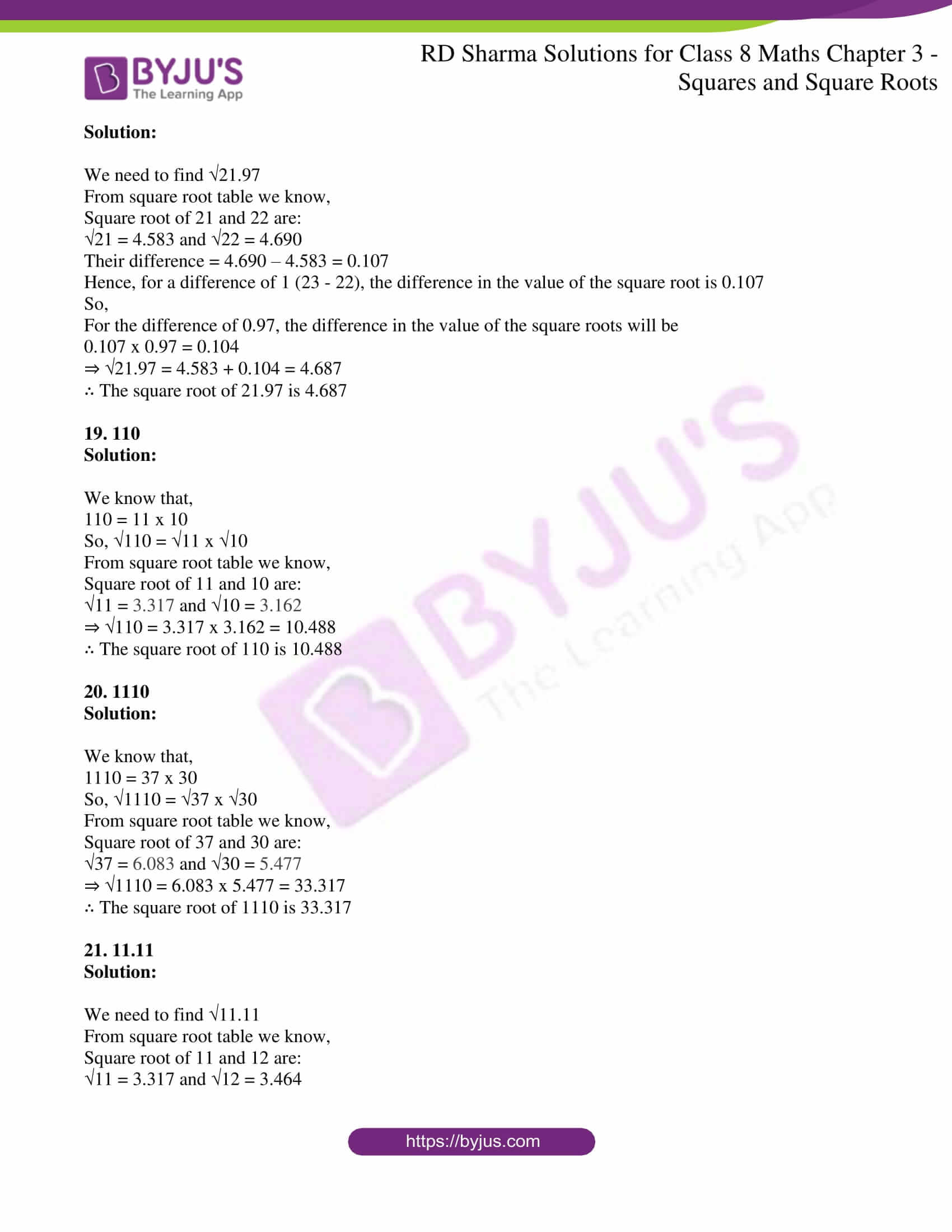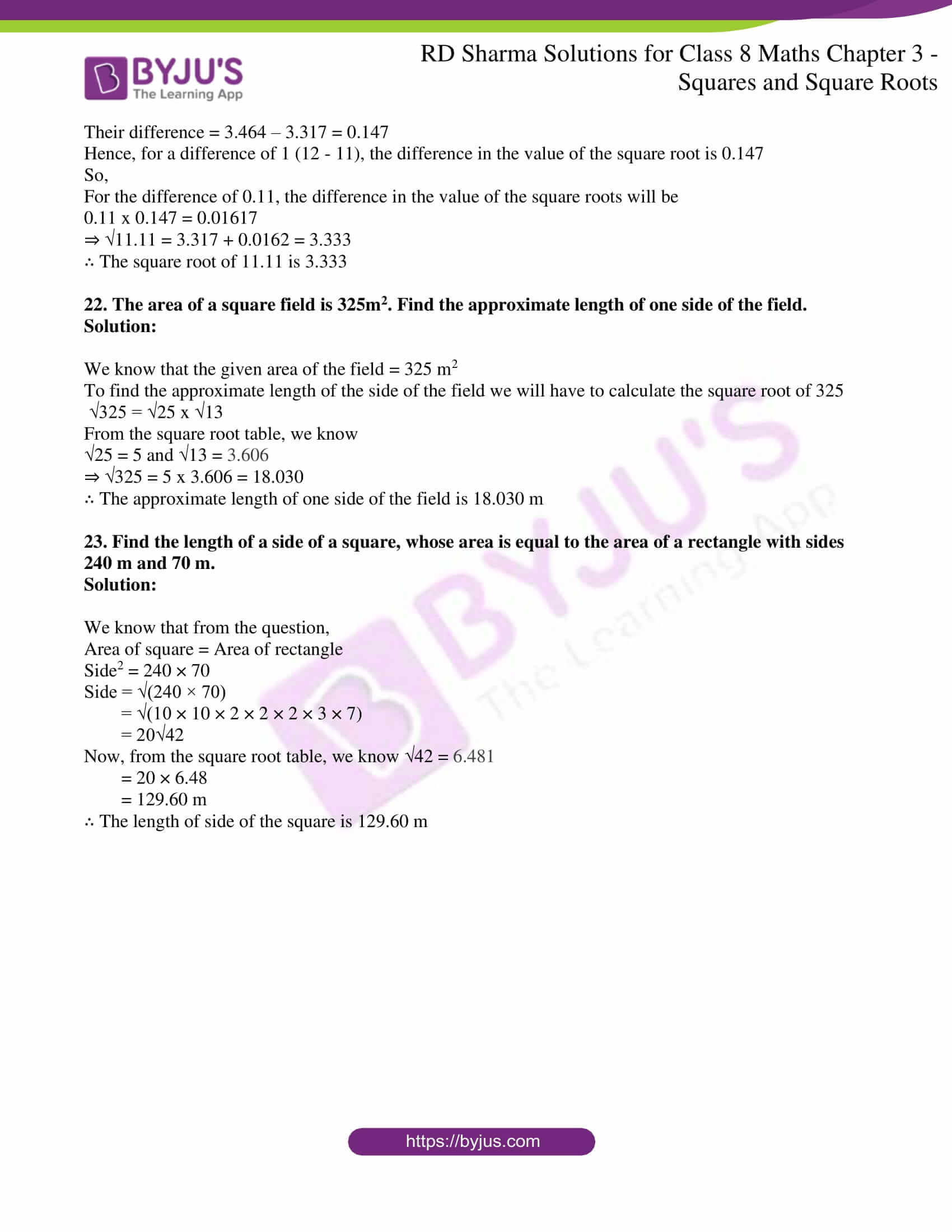### Access Answers to RD Sharma Solutions for Class 8 Maths Exercise 3.9 Chapter 3 Squares and Square Roots

#### EXERCISE 3.9 PAGE NO: 3.61

 Square Root Table Number Square Root(√) Number Square Root(√) Number Square Root(√) Number Square Root(√) Number Square Root(√) 1 1 21 4.583 41 6.403 61 7.81 81 9 2 1.414 22 4.69 42 6.481 62 7.874 82 9.055 3 1.732 23 4.796 43 6.557 63 7.937 83 9.11 4 2 24 4.899 44 6.633 64 8 84 9.165 5 2.236 25 5 45 6.708 65 8.062 85 9.22 6 2.449 26 5.099 46 6.782 66 8.124 86 9.274 7 2.646 27 5.196 47 6.856 67 8.185 87 9.327 8 2.828 28 5.292 48 6.928 68 8.246 88 9.381 9 3 29 5.385 49 7 69 8.307 89 9.434 10 3.162 30 5.477 50 7.071 70 8.367 90 9.487 11 3.317 31 5.568 51 7.141 71 8.426 91 9.539 12 3.464 32 5.657 52 7.211 72 8.485 92 9.592 13 3.606 33 5.745 53 7.28 73 8.544 93 9.644 14 3.742 34 5.831 54 7.348 74 8.602 94 9.695 15 3.873 35 5.916 55 7.416 75 8.66 95 9.747 16 4 36 6 56 7.483 76 8.718 96 9.798 17 4.123 37 6.083 57 7.55 77 8.775 97 9.849 18 4.243 28 6.164 58 7.616 78 8.832 98 9.899 19 4.359 29 6.245 59 7.681 79 8.888 99 9.95 20 4.472 40 6.325 60 7.746 80 8.944 100 10

Using square root table, find the square roots of the following:

1. 7

Solution:

From square root table we know,

Square root of 7 is:

√7 = 2.645

∴ The square root of 7 is 2.645

2. 15

Solution:

We know that,

15 = 3 x 5

So, √15 = √3 x √5

From square root table we know,

Square root of 3 and 5 are:

√3 = 1.732 and √5 = 2.236

⇒ √15 = 1.732 x 2.236 = 3.873

∴ The square root of 15 is 3.873

3. 74

Solution:

We know that,

74 = 2 x 37

So, √74 = √2 x √37

From square root table we know,

Square root of 2 and 37 are:

√2 = 1.414 and √37 = 6.083

⇒ √74 = 1.414 x 6.083 = 8.602

∴ The square root of 74 is 8.602

4. 82

Solution:

We know that,

82 = 2 x 41

So, √82 = √2 x √41

From square root table we know,

Square root of 2 and 41 are:

√2 = 1.414 and √41 = 6.403

⇒ √82 = 1.414 x 6.403 = 9.055

∴ The square root of 82 is 9.055

5. 198

Solution:

We know that,

198 = 2 x 9 x 11

So, √198 = √2 x √9 x √11

From square root table we know,

Square root of 2, 9 and 11 are:

√2 = 1.414, √9 = 3 and √11 = 3.317

⇒ √198 = 1.414 x 3 x 3.317 = 14.071

∴ The square root of 198 is 14.071

6. 540

Solution:

We know that,

540 = 6 x 9 x 10

So, √540 = √6 x √9 x √10

From square root table we know,

Square root of 6, 9 and 10 are:

√6 = 2.449, √9 = 3 and √10 = 3.162

⇒ √540 = 2.449 x 3 x 3.162 = 23.24

∴ The square root of 540 is 23.24

7. 8700

Solution:

We know that,

8700 = 87 x 100

So, √8700 = √87 x √100

From square root table we know,

Square root of 87 and 100 are:

√8700 = 9.327 and √100 = 10

⇒ √8700 = 9.327 x 10 = 93.27

∴ The square root of 8700 is 93.27

8. 3509

Solution:

We know that,

3509 = 121 x 29

So, √3509 = √121 x √29

From square root table we know,

Square root of 121 and 29 are:

√121 = 11 and √29 = 5.385

⇒ √3509 = 11 x 10 = 5.385

∴ The square root of 3509 is 59.235

9. 6929

Solution:

We know that,

6929 = 169 x 41

So, √6929 = √169 x √41

From square root table we know,

Square root of 169 and 41 are:

√169 = 13 and √41 = 6.403

⇒ √6929 = 13 x 6.403 = 83.239

∴ The square root of 6929 is 83.239

10. 25725

Solution:

We know that,

25725 = 3 x 7 x 25 x 49

So, √25725 = √3 x √7 x √25 x √49

From square root table we know,

Square root of 3, 7, 25 and 49 are:

√3 = 1.732, √7 = 2.646, √25 = 5 and √49 = 7

⇒ √25725 = 1.732 x 2.646 x 5 x 7 = 160.41

∴ The square root of 25725 is 160.41

11. 1312.

Solution:

We know that,

1312 = 2 x 16 x 41

So, √1312 = √2 x √16 x √41

From square root table we know,

Square root of 2, 16 and 41 are:

√2 = 1.414, √16 = 4 and √41 = 6.403

⇒ √1312 = 1.414 x 4 x 6.403 = 36.22

∴ The square root of 1312 is 36.22

12. 4192

Solution:

We know that,

4192 = 2 x 16 x 131

So, √4192 = √2 x √16 x √131

From square root table we know,

Square root of 2 and16 are:

√2 = 1.414 and √16 = 4

The square root of 131 is not listed in the table

Thus, let’s apply long division to find it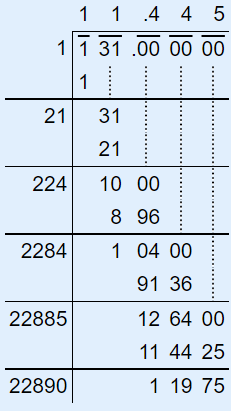So, square root of 131 is 11.445

Now,

⇒ √4192 = 1.414 x 4 x 11.445 = 64.75

∴ The square root of 4192 is 64.75

13. 4955

Solution:

We know that,

4955 = 5 x 991

So, √4955 = √5 x √991

From square root table we know,

Square root of 5 is:

√5 = 2.236

The square root of 991 is not listed in the table

Thus, let’s apply long division to find it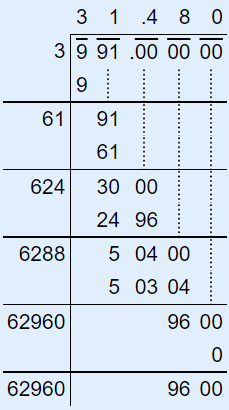So, square root of 991 is 31.480

Now,

⇒ √4955 = 2.236 x 31.480 = 70.39

∴ The square root of 4955 is 70.39

14. 99/144

Solution:

We know that,

99/144 = (9 x 11) / (12 x 12)

So, √(99/144) = √[(9 x 11) x (12 x 12)]

= 3/12 x √11

From square root table we know,

Square root of 11 is:

√11 = 3.317

⇒ √(99/144) = 3/12 x 3.317 = 3.317/4 = 0.829

∴ The square root of 99/144 is 0.829

15. 57/169

Solution:

We know that,

57/169 = (3 x 19) / (13 x 13)

So, √(57/169) = √[(3 x 19) x (13 x 13)]

= √3 x √19 x 1/13

From square root table we know,

Square root of 3 and 19 is:

√3 = 1.732 and √19 = 4.359

⇒ √(57/169) = 1.732 x 4.359 x 1/13 = 0.581

∴ The square root of 57/169 is 0.581

16. 101/169

Solution:

We know that,

101/169 = 101 / (13 x 13)

So, √(101/169) = √[101 / (13 x 13)]

= √101/13

From square root table we don’t have the square root of 101

Thus, we have to manipulate the number such that we get the square root of a number less than 100

√101 = √(1.01 x 100)

= √1.01 x 10

Now, we have to find the square of 1.01

We know that,

√1 = 1 and √2 = 1.414 (From the square root table)

Their difference = 1.414 – 1 = 0.414

Hence, for a difference of 1 (2 – 1), the difference in the value of the square root is 0.414

So,

For the difference of 0.01, the difference in the value of the square roots will be

0.01 x 1.414 = 0.00414

∴√1.01 = 1 + 0.00414 = 1.00414

Then, √101 = 1.00414 x 10 = 10.0414

⇒ √(101/169) = √101/13 = 10.0414/13

∴ The square root of 101/169 is 0.773

17. 13.21

Solution:

We need to find √13.21

From square root table we know,

Square root of 13 and 14 are:

√13 = 3.606 and √14 = 3.742

Their difference = 3.742 – 3.606 = 0.136

Hence, for a difference of 1 (14 – 13), the difference in the value of the square root is 0.136

So,

For the difference of 0.21, the difference in the value of the square roots will be

0.136 x 0.21 = 0.0286

⇒ √13.21 = 3.606 + 0.0286 = 3.635

∴ The square root of 13.21 is 3.635

18. 21.97

Solution:

We need to find √21.97

From square root table we know,

Square root of 21 and 22 are:

√21 = 4.583 and √22 = 4.690

Their difference = 4.690 – 4.583 = 0.107

Hence, for a difference of 1 (23 – 22), the difference in the value of the square root is 0.107

So,

For the difference of 0.97, the difference in the value of the square roots will be

0.107 x 0.97 = 0.104

⇒ √21.97 = 4.583 + 0.104 = 4.687

∴ The square root of 21.97 is 4.687

19. 110

Solution:

We know that,

110 = 11 x 10

So, √110 = √11 x √10

From square root table we know,

Square root of 11 and 10 are:

√11 = 3.317 and √10 = 3.162

⇒ √110 = 3.317 x 3.162 = 10.488

∴ The square root of 110 is 10.488

20. 1110

Solution:

We know that,

1110 = 37 x 30

So, √1110 = √37 x √30

From square root table we know,

Square root of 37 and 30 are:

√37 = 6.083 and √30 = 5.477

⇒ √1110 = 6.083 x 5.477 = 33.317

∴ The square root of 1110 is 33.317

21. 11.11

Solution:

We need to find √11.11

From square root table we know,

Square root of 11 and 12 are:

√11 = 3.317 and √12 = 3.464

Their difference = 3.464 – 3.317 = 0.147

Hence, for a difference of 1 (12 – 11), the difference in the value of the square root is 0.147

So,

For the difference of 0.11, the difference in the value of the square roots will be

0.11 x 0.147 = 0.01617

⇒ √11.11 = 3.317 + 0.0162 = 3.333

∴ The square root of 11.11 is 3.333

22. The area of a square field is 325m2. Find the approximate length of one side of the field.

Solution:

We know that the given area of the field = 325 m2

To find the approximate length of the side of the field we will have to calculate the square root of 325

√325 = √25 x √13

From the square root table, we know

√25 = 5 and √13 = 3.606

⇒ √325 = 5 x 3.606 = 18.030

∴ The approximate length of one side of the field is 18.030 m

23. Find the length of a side of a square, whose area is equal to the area of a rectangle with sides 240 m and 70 m.

Solution:

We know that from the question,

Area of square = Area of rectangle

Side2 = 240 × 70

Side = √(240 × 70)

= √(10 × 10 × 2 × 2 × 2 × 3 × 7)

= 20√42

Now, from the square root table, we know √42 = 6.481

= 20 × 6.48

= 129.60 m

∴ The length of side of the square is 129.60 m

## RD Sharma Solutions for Class 8 Maths Exercise 3.9 Chapter 3 – Squares and Square Roots

Exercise 3.9 of RD Sharma Solutions for Chapter 3 Squares and Square Roots, this exercise mainly deals with the concepts related to finding the approximate values of square roots by using square root tables. The students can solve problems of RD Sharma textbook for Class 8 using the solutions as reference material. This mainly improves confidence among students in solving tricky and lengthy problems easily. The PDF is easily accessible by the students which help in speeding up their exam preparation.